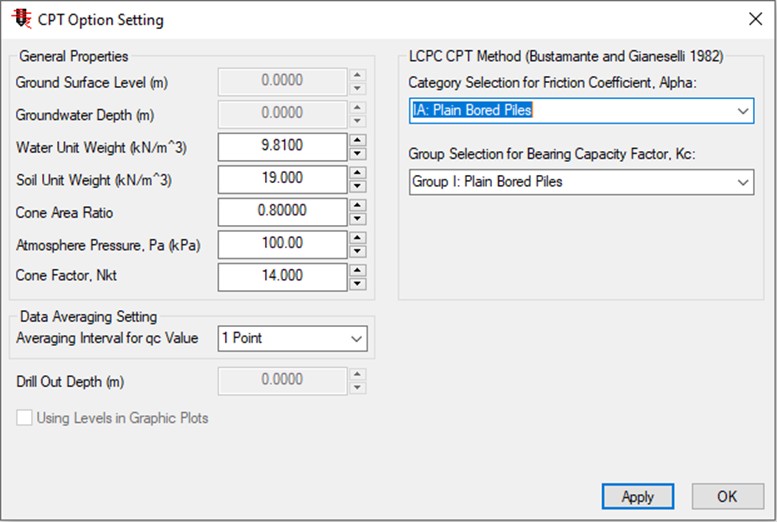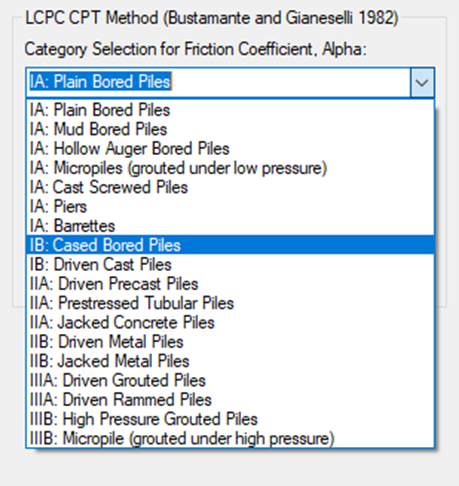top of page

# Importing CPT data into the PileAXL program for calculating axial capacity and settlement of piles

In this blog, we explore the capabilities of PileAXL program in analyzing the axial capacity and settlement of piles, by showcasing how to import cone penetration test (CPT) data into the software. CPTu manager dialog shown in the figure below can be opened by clicking the submenu of “CPT Data Option” under “Define” main menu or clicking toolbar button of “Import CPT Data” of the program.The type of CPT data file can be selected by the users before the CPT data is imported. Currently, the following file types and options are supported in the program:

• Read data from text file (*.TXT file)

• Read data from DAT file (*.DAT file)

• Read data from GEF file (*.GEF file)

• Read data from CSV file (*.CSV file)

• Read data from COR file (*.COR file)The following dialog of importing CPT raw data will appear when the “Next” button is clicked. It shows the data structure of the CPT file before it is imported into the program. The section of “File Preview” presents a preview of the selected CPT file.The column of “Line” shows the number of the line. The column of “CPT Data” shows the CPT data content for the associated line. The bottom section of the dialog is “Data Structure” which can be used to define the input items (Depth, qc, fs, and u2) and units (m, kPa or MPa).

Read the CPT data from the line number” section defines the line number from which the CPT raw data should be imported. The line number will need to be selected by the users correctly in order to import the right data block. The line number is usually the first line of the measured CPT data. “Total line number of the input data” section shows the number of CPT data line. It is read-only and cannot be changed.After all the settings are completed, click on “Import” button to import the selected CPT raw data. This will update the dialog of CPT File Manager with the information of CPT file name. The button of “Display” will become active if the CPT file is imported successfully. If the users click this button, then a CPT plot dialog will be opened and the imported CPT results will be displayed on the graphical area, as shown in the figure below.After importing the CPT data, the users are also able to edit the CPT data and change the CPT file name if required and this can be achieved by clicking the button of “Edit” from CPT Manager dialog. The following figure shows the dialog for editing the import CPT data.Only one CPT file can be used in the pile axial capacity and settlement analysis although multiple CPT files can be imported into the program. The CPT file can be selected from the dropdown menu under the title of “Select CPT file for pile capacity and settlement analysis” from the CPT file manager dialog.

Note that the selected CPT data file will be used in the analysis only if the soil layers include the material set which calculates the ultimate shaft resistance and end bearing resistance using the cone tip resistance (qc) value. The imported CPT data will have no effects on the material sets and where the cone tip resistance (qc) value is not used for axial capacity calculation. When the option of “None” is selected from the dropdown menu, CPT data file will not be used in the pile axial capacity and settlement analysis.

The following figure shows the profile of CPT data in the graphic area of the main program.More setting parameters can be input and changed by the users using “Display Option” dialog. The color and the maximum Qc value of CPT profile can be updated by the users from this dialog.The CPT manager tool built into the PileAXL program also has some basic functions of ALLCPT program which is another software product developed by Innovative Geotechnics Pty Ltd. A right click of the mouse on the main CPT plot as shown in the figure below will invoke a floating menu with four different menu items. There are four different sub-menus which can be selected by the users as below:

• Display CPT Data Table

• Select Plot Option

• Copy CPT Plot

• Print CPT PlotClicking the submenu of “Display CPT Data Table” opens the table as shown in the figure below. All the imported CPT data together with various interpreted soil parameters are presented into the table form.More plotting options can be selected by clicking the submenu of “Select Plot Option”. The following figure shows the plot options which are available to the users. A number of soil parameters calculated from the imported CPT data can be selected by the users.The figure below shows a typical plot with different selected items such as Young’s modulus (Es), soil behaviour type number (SBTn), effective friction angle (ɸ’), soil behaviour type index (Ic) and the equivalent SPT-N values calculated from the imported CPT data.For the imported CPT files, the users can define the specific options which are used by the program to process and interpret the CPT data. The figure below shows the dialog for CPT option setting which can be opened by clicking the button of “Option” as shown in the CPT file manager dialog.The following parameters for CPT data interpretation can be defined by the users:

• Ground surface level. Ground surface level measured at the location of CPT testing can be input into the program. The ground surface level will be then used in the graphic plots when the option of “Using Levels in Graphic Plots” is checked. By default, all graphic plots in PileAXL program are presented in terms of depth.

• Groundwater depth. The groundwater depth can be input into the program to calculate the groundwater pressure. The default value is 0.

• Water unit weight. The water unit weight can be input into the program for various CPT correlation calculations. The default value is 9.81 kN/m3.

• Soil unit weight. The soil unit weight can be input into the program by the users to calculate the total vertical stress. The default value is 19 kN/m3.

• Cone area ratio. The cone area ratio can be input into the program by the users and the default value is 0.8.

• Atmosphere pressure. The atmosphere pressure can be input into the program by the users and the default value is 100 kPa.

• Cone factor. Cone factor can be input into the program by the users for the calculation of undrained shear strength and the default value is 14.

• Averaging interval for qc value. This option allows the users to choose the averaging interval value for the measured qc values. The default selection is 1 point which means that no averaging process will be undertaken for the measured qc values.

• Using levels in graphic plots. When this option is checked, all CPT graphic plots will be presented in terms of elevation instead of depth.

The following parameters for LCPC CPT method can be defined by the users:

• Category selection for friction coefficient, Alpha. The pile types available for selection is shown in the figure below.• Group selection for bearing capacity factor, Kc. The pile types available for selection is shown in the figure below.91 views